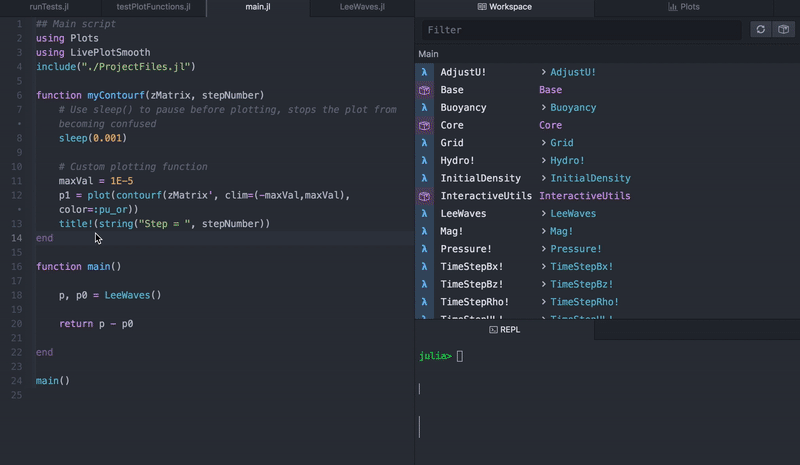## SmoothLivePlot.jl

A Julia package for creating live-style plots during calculations.
Author williamjsdavis
Popularity
24 Stars
Updated Last
10 Months Ago
Started In
March 2020

# SmoothLivePlot.jl

`SmoothLivePlot.jl` is a Julia package for creating live-style plots during calculations.

# Motivation

Updating the Juno plot plane during calculations creates new plots on top of the old ones. This produces a flickering effect e.g.:

To smoothly update of plots, I generalised a solution found by user ckneale. It uses Observables.jl and WebIO.jl so that the plot can listen to changes in its elements.

Currently, I have tested the following capabilities:

• Modifying values in X and/or Y array(s) in scatter and plot
• Modifying colours in scatter and plot
• Modifying text elements (e.g. titles, xlabels, etc...)
• Modifying matricies in contour plots
• Adding new elements to X,Y arrays in 2d line and scatter plots
• Adding new elements to X,Y,Z in 3d line and scatter plots

Note: this package is designed to work with the plot plane in Juno. If you force it to plot in a gui it will look really weird.

# Using the package

1. Import the module using `using SmoothLivePlot`.
2. Create a live plot with macro `outPlotObject = @makeLivePlot myPlotFunction(argument1, argument2, ...)`.
• Function `myPlotFunction(argument1, argument2, ...)` is a user defined plotting function.
• Output `outPlotObject` is a mutable output array of plot features. Its elements are the input aruments of `myPlotFunction()`.
3. Modify plot elements with function `modifyPlotObject!(outPlotObject, arg2 = newArg2, arg1 = newArg1, ...)`.
• The first argment of `modifyPlotObject!()` must be the mutable output array.
• The following argments are optional and named. The name/value pair must be `arg<x> = newArg1`, where `<x>` in the name is an integer that indicates the position of the argument in the original plotting function `myPlotFunction()`.
• E.g. to modify `argument2` to `newArgument2`, use `modifyPlotObject!(outPlotObject, arg2 = newArgument2)`.
• The modified arguments do not have to be in any specific order, and are updated at the same time.

### Short example

Here's a video showing an output live-plot from some magnetohydrodynamic calculations: